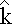Magnetic Field from a Long Straight Wire

Note that, to plot a field line, double-click on one or more points that are not on the wire.

For a straight wire we just derived that the magnetic field is:

B = ( μo I / 4π a ) [cos(θ1) - cos(θ2]This simplifies nicely in the limit of an infinitely long wire, where θ1 = 0 and θ2 = 180:

B = ( μo I / 2π r )where r represents the distance from the wire to the point where the field is being determined.

For an infinitely long straight wire, the magnetic field lines are circles centered on the wire. The direction is given by a right-hand rule:

With your right hand, point your thumb in the direction of the current. When you curl your fingers they curl in the direction of the magnetic field.